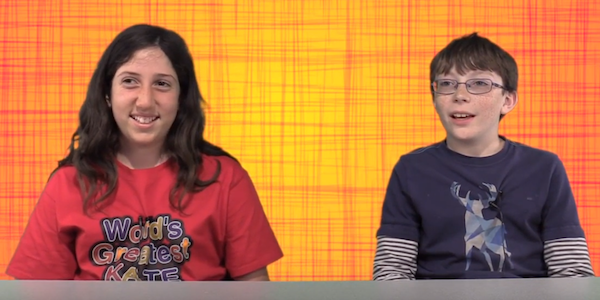# Proportional Reasoning Unit

Students work with an applet that allows them to run races between two cars by entering values for each car’s distance and time. They explore the effect on the cars’ speeds by first working with one quantity and then changing both distance and time. Through a series of diagram-construction activities, they form a ratio, iterate and split ratios, and create a unit ratio. Students move from reasoning additively to forming multiplicative comparisons.

##### Lesson 1: Changing One Quantity at a Time

Kate and Christopher begin to make sense of proportional reasoning in a speed context.  They use an applet called Races to explore how to make one car go slower or faster than another car.

##### Lesson 2: Changing Two Quantities

Kate and Christopher use the Races applet to figure out how to make two cars go the same speed when the cars travel different amounts of distance and time.

##### Lesson 3: Creating Diagrams to Represent Ratios

Kate and Christopher create a diagram to explain why the distance and times values for two cars make them go the same speed. In the process, they form a ratio by joining the distance and time values for one car into a unit or “little trip,” which can be repeated (iterated) or split apart (partitioned) to solve proportional reasoning problems.

##### Lesson 4: Using Diagrams to Iterate Ratios

Kate and Christopher discover a way to solve proportional reasoning problems in a speed context, by drawing diagrams and repeating (iterating) a ratio of distance and time.

##### Lesson 5: Forming a Unit Ratio

Kate and Christopher extend their use of diagrams to form a unit ratio in a speed context.

##### Lesson 6: Solving Proportions Using Multiplication and Division

Kate and Christopher make connections between their speed diagrams and the operations of multiplication and division.  This helps give meaning to number sentences used to solve proportion problems.

##### Lesson 7: Making Multiplicative Comparisons

Kate and Christopher use an applet called Making Pink Paint to solve proportional reasoning problems. They choose amounts of red and white paint to make two batches of paint that are the same shade of pink. In the process, they form ratios by comparing amounts of white paint to red paint multiplicatively.